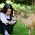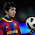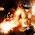This blog is under construction

Tuesday, 9 July 2013

C program to calculate correlation coefficient

How to find correlation coefficient?
Please check the below link to get better understanding on correlation coefficient.

Write a C program to calculate correlation coefficient.

#include <stdio.h>
#include <math.h>

int main() {
int x, y, xy, xsquare, ysquare;
int i, n, xsum, ysum, xysum, xsqr_sum, ysqr_sum;
float coeff, num, deno;

xsum = ysum = xysum = xsqr_sum = ysqr_sum = 0;

/* get the number of entries from the user */
printf("Enter the value for n:");
scanf("%d", &n);

/* get the values for x and y  from the user */
printf("Enter the value for x and y:\n");
for (i = 0; i < n; i++) {
printf("x[%d] and y[%d]: ", i, i);
scanf("%d%d", &x[i], &y[i]);
}

/* find the needed data to manipulate correlation coeff */
for (i = 0; i < n; i++) {
xy[i] = x[i] * y[i];
xsquare[i] = x[i] * x[i];
ysquare[i] = y[i] * y[i];
xsum = xsum + x[i];
ysum = ysum + y[i];
xysum = xysum + xy[i];
xsqr_sum = xsqr_sum + xsquare[i];
ysqr_sum = ysqr_sum + ysquare[i];
}

num = 1.0 * ((n * xysum) - (xsum * ysum));
deno = 1.0 * ((n * xsqr_sum - xsum * xsum)* (n * ysqr_sum - ysum * ysum));

/* calculate correlation coefficient */
coeff = num / sqrt(deno);

/* print the result */
printf("Correlation Coefficient : %.4f\n", coeff);
return 0;
}

Note:
gcc corr_coeff.c -lm => linked math library since we have used sqrt() math function.

Output:
jp@jp-VirtualBox:~/\$ gcc corr_coeff.c -lm
jp@jp-VirtualBox:~/\$ ./a.out
Enter the value for n:6
Enter the value for x and y:
x and y: 43 99
x and y: 21 65
x and y: 25 79
x and y: 42 75
x and y: 57 87
x and y: 59 81
Correlation Coefficient : 0.5298

1.Programming is combination of intelligent and creative work. Programmers can do anything with code. The entire Programming tutorials that you mention here on this blog are awesome. Beginners Heap also provides latest tutorials of Programming from beginning to advance level.
Be with us to learn programming in new and creative way.

2.Very informative article.Thank you author for posting this kind of article .

http://www.wikitechy.com/view-article/power-function-in-c-with-example-and-explanation

Both are really good,
Cheers,
Venkat

3.This comment has been removed by the author.

4.This comment has been removed by the author.

5.This comment has been removed by the author.